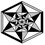# Guessed correct but don't know the solution

I attempted a question which says:

$f(x)=3x^3 +2x^2 +14x -5.$

a = sum of real roots. Find 93a. I guessed the answer to be 31 and it was correct. Can anyone help me to solve it actually?

I have one more question. Can't we view the solutions of the practice problems we are unable to solve?Note by Maharnab Mitra
6 years, 9 months ago

This discussion board is a place to discuss our Daily Challenges and the math and science related to those challenges. Explanations are more than just a solution — they should explain the steps and thinking strategies that you used to obtain the solution. Comments should further the discussion of math and science.

When posting on Brilliant:

• Use the emojis to react to an explanation, whether you're congratulating a job well done , or just really confused .
• Ask specific questions about the challenge or the steps in somebody's explanation. Well-posed questions can add a lot to the discussion, but posting "I don't understand!" doesn't help anyone.
• Try to contribute something new to the discussion, whether it is an extension, generalization or other idea related to the challenge.

MarkdownAppears as
*italics* or _italics_ italics
**bold** or __bold__ bold
- bulleted- list
• bulleted
• list
1. numbered2. list
1. numbered
2. list
Note: you must add a full line of space before and after lists for them to show up correctly
paragraph 1paragraph 2

paragraph 1

paragraph 2

[example link](https://brilliant.org)example link
> This is a quote
This is a quote
    # I indented these lines
# 4 spaces, and now they show
# up as a code block.

print "hello world"
# I indented these lines
# 4 spaces, and now they show
# up as a code block.

print "hello world"
MathAppears as
Remember to wrap math in $$ ... $$ or $ ... $ to ensure proper formatting.
2 \times 3 $2 \times 3$
2^{34} $2^{34}$
a_{i-1} $a_{i-1}$
\frac{2}{3} $\frac{2}{3}$
\sqrt{2} $\sqrt{2}$
\sum_{i=1}^3 $\sum_{i=1}^3$
\sin \theta $\sin \theta$
\boxed{123} $\boxed{123}$

Sort by:

Notice that: $3x^3 + 2x^2 + 14x -5 \equiv (3x-1)(x^2+x+5)$ Consider the nature of the roots of $x^2 + x + 5$. We will use the discriminant for this: $1^2- 4 \times 1 \times 5 = -19 < 0$ Therefore, it is clear the $x^2 + x + 5$ has no real roots. From this we can see that the only real root is when: $3x - 1 = 0 \Rightarrow x = \frac{1}{3}$ Therefore: $a = \frac{1}{3} \Rightarrow 93a = \fbox{31}$

- 6 years, 9 months ago

Thanks!

- 6 years, 9 months ago

I can't help with the question but I am curious as to how did you even guess that?

- 6 years, 9 months ago

Maharnab must have guessed it as question was positive integer type. 93a is asked means a might not be integer but 93a must be. So factors of 93 are limited...

- 6 years, 9 months ago

Is this a question included in the Practice section?

- 6 years, 9 months ago

Yes, it is. Is its solution available at Brilliant.org?

- 6 years, 9 months ago

On the page for the question on the top right there is a grey icon like two chain links connected, like the on the bottom right of the original post. If you put the mouse on it it says "copy URL". Click copy and paste it into the website search bar. It should go to another page with the same problem and a button with reveal solution on it.

- 6 years, 9 months ago

@C D I did this and now I'm stuck on one of my algebra topics and can't progress! :(

- 6 years, 9 months ago

Darn! Which topic did you encounter this bug in?

Staff - 6 years, 9 months ago

Polynomial inequalities 1. The 'next' button seems to have been removed and now there's just nothing (I believe it would happen for any problem within the practice sections however)

- 6 years, 9 months ago

Just to say, every time I did this it worked but a glitch or something stops you from doing that problem set again. If you click "Next Problem" it doesn't work. I hope they fix it soon.

- 6 years, 9 months ago

@C D Which topic are you experiencing this glitch in?

Staff - 6 years, 9 months ago

Staff - 6 years, 9 months ago

- 6 years, 9 months ago

Can you give the link to it?

- 6 years, 9 months ago

We can see here that $\frac{1}{3}$ is a zero of the polynomial.Then we can divide the polynomial by $3x -1$ and get the quadratic polynomial $x^{2}+x+5$, which is having all its zeroes imaginary.Hence, we get the result.

- 6 years, 9 months ago

If certain fractions are the zeros of a polynomial, is there a better way to find them other than by observing and performing trials?

- 6 years, 9 months ago

since the cubic polynomial may have 3 real roots or 1 real root but never 2 real roots as in that case the rest complex root will not have any conjugate pair left. now for three real roots we get a= (-2/3) i.e
93a= -62 which is impossible as the answer must be a positive integer.[just for checking] and then being convinced with the fact that only one real root is possible i used the factorisation method same as OLIVER W. and finally got the answer 93a= 31

- 6 years, 9 months ago

graphically we can prove that a=1/3

- 6 years, 9 months ago

Isn't there a cubic formula to get the roots of a cube without trial and error?

- 6 years, 9 months ago

I'm just curious is there any formula or something that can directly compute the value of x in this like the quadratic equation ?

- 6 years, 9 months ago